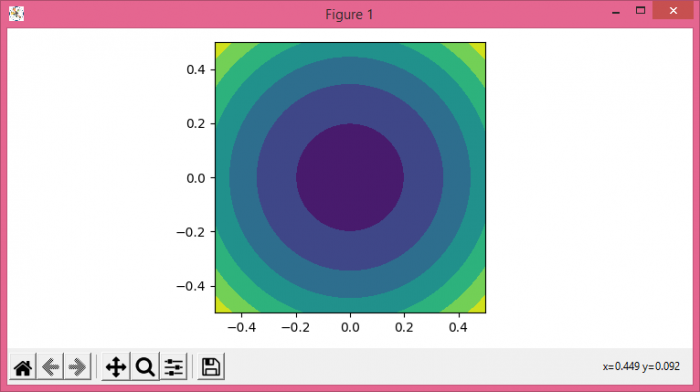# How to make a circular matplotlib.pyplot.contourf?

To make a circular matplotlib.pyplot.contourf, we can take the following steps −

• Set the figure size and adjust the padding between and around the subplots.

• Create x, y, a, b and c data points using Numpy.

• Create a figure and a set of subplots.

• Make a Contour plot using contourf() method.

• Set the aspect ratios.

• To display the figure, use show() method.

## Example

import numpy as np
import matplotlib.pyplot as plt

plt.rcParams["figure.figsize"] = [7.50, 3.50]
plt.rcParams["figure.autolayout"] = True

x = np.linspace(-0.5, 0.5, 100)
y = np.linspace(-0.5, 0.5, 100)

a, b = np.meshgrid(x, y)
c = a ** 2 + b ** 2 - 0.2

figure, axes = plt.subplots()

axes.contourf(a, b, c)

axes.set_aspect(1)

plt.show()

## OutputUpdated on: 10-Aug-2021

462 Views